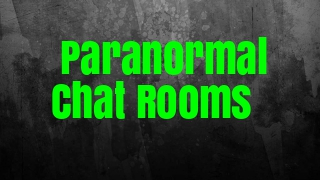Mystic Sisterhood

Spiritual, Paranormal & Natural Science Forums### Free Psychic & Paranormal Chat Rooms### Next Chat Event### ..# Numerology CalculatorCloudZodiac :Posts : 3984
Points : 9572
Times User Thanked: : 177
Join date : 2018-01-12

How to Calculate Your Life Path Number

2.Do not reduce the Master Numbers of 11 or 22 to single digits until the final calculation.

Sound Simple? It is!

Part 1: Grouping The Numbers Together

There are 2 ways to group the numbers, the correct way, and the incorrect way.

Let’s point out the incorrect way first since we see this confusing people. The incorrect way is to "just add everything together".

First let’s look at how people most often incorrectly calculate life path numbers. Why are we showing the incorrect way first? It's easier to learn what not to do followed by what to do.

The Incorrect Way

Birth Date: May 4, 1977

May = 5

4 = 4

1977 = 1977

(5 + 4 + 1 + 9 + 7 + 7) = 33

At which point people proclaim, "I'm a Master Number 33!"

But this is incorrect.

The Correct Way

The correct way to calculate the Life Path Number is to group the Month, Day, and Year, and add them individually, reduce to a single number for each, then reduce them to a single number.

For example:

Birth Date: May 4, 1977

May = 5

4 = 4

1977 = (1 + 9 + 7 + 7) = 24 = (2 + 4) = 6

Then add the totals from each above group

(5 + 4 + 6) = 15 = (1 + 5) = 6

Correct: Life Path 6

Note that the year in the above calculation is reduced to a single number before it is added to the other numbers from the Month and Day.

In some situations the final Life Path number will come out the same when done correctly and incorrectly, this is why it is important to do it the correct way all the time.

Here’s another example using 2 digit Month and Day:

Birth Date: December 21, 1954

December = 12 = (1 + 2) = 3

21 = (2 + 1) = 3

1954 = (1 + 9 + 5 + 4) = 19 = (1 + 9) = 10 = (1 + 0) = 1

(3 + 3 + 1) = 7

Correct: Life Path 7Zodiac :Posts : 32